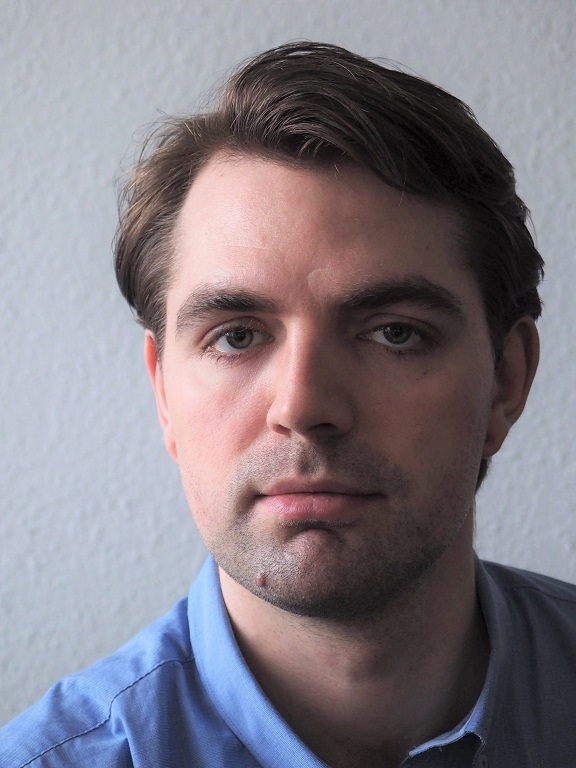# Tobias Kasper Skovborg RitschelTobias K. S. Ritschel Postdoctoral Researcher Department of Applied Mathematics and Computer Science Technical University of Denmark DK-2800 Kgs. Lyngby Denmark

Tobias K. S. Ritschel
DTU Compute – Department of Applied Mathematics and Computer Science
Technical University of Denmark

Building 303B, office 121
Matematiktorvet
DK-2800 Kgs. Lyngby
Denmark

Tel: +45 6062 1486
E-mail: tobk@dtu.dk

## Core competences

Algorithms:
Model predictive control | Optimal control | Maximum likelihood estimation | State estimation | Model order reduction | Numerical optimization | Numerical simulation

Mathematical modeling:
Stochastic differential equations | Partial differential equations | Differential-algebraic equations | Ordinary differential equations

Applications:
Diabetes | Oil and gas (phase equilibrium systems) | Power grids | Bioreactors | Epidemiology

Programming:
C/C++ | Matlab | Java | Android | Python | C#